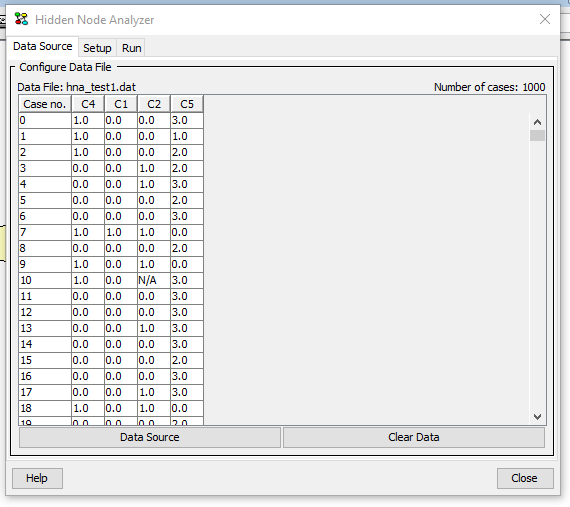# Hidden Node Analyzer¶

The Hidden Node Analyzer is a wizard for determining the best number of states of a (hidden) discrete chance node in a Bayesian network from a dataset. The Hidden Node Analyzer has three steps:

• selecting the hidden node and setting the parameters for identification of the number of states of the hidden node

• the analysis

Each step is described in the following sections.

To perform any analysis the wizard will first need a set of cases. Cases can be imported by loading a HUGIN data file or connecting to a database service and import. Click on button “Data Source” to import data.

After importing a set of cases, the Data Source pane will display a table with the imported cases, see Figure 1. To discard all imported cases click on the button “Clear Data”.Figure 1: Data Source pane. A set of cases has been imported.¶

## Setting Parameters¶

The Hidden Node Analyzer can be used to identify the number of states of a hidden node. A node is hidden if no case in the dataset contains an observation on the node.

To identify the number of states of a hidden node, the user should specify the hidden node, the minimum number of states, the maximum number of states, and whether the conditional probability table (CPT) of the hidden node should be randomized (default option) as illustrated in Figure 2.

The Hidden Node Analyzer supports discrete chance nodes only and only if there are atleast one non-boolean node.

## Analysis¶

The Hidden Node Analyzer iterates over the possible state space sizes of the hidden from minimum to maximum as selected by the user. In each iteration an EM learning operation is performed from the initial network, i.e., the state of the network when the wizard was opened. The conditional probability table of the hidden node is randomized, if the user has selected this option (default). For each iteration the AIC, BIC and log-likelihood are displayed to the user.

Notice that to perform the analysis, the network must be compiled and one EM learning operation is performed for each state considered. This may result in long running times.

Figure 3 shows the result of running the Hidden Node Analyzer on an example network and dataset.